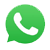Power-Torque

Torque (lb.in) = 63,025 x Power (HP) / Speed (RPM)

Power (HP) = Torque (lb.in) x Speed (RPM) / 63,025

Torque (N.m) = 9.5488 x Power (kW) / Speed (RPM)

Power (kW) = Torque (N.m) x Speed (RPM) / 9.5488

 Torque from power
 Enter Power : HPkW Enter Speed : RPM Torque : lb.inN.m

 Power from torque
 Enter Torque : lb.inN.m Enter Speed : RPM Power : HPkW